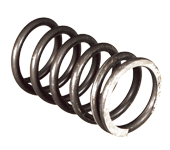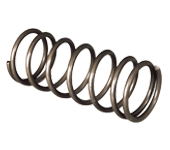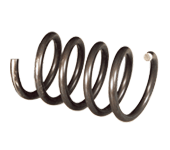Compression springs

How do you design a compression spring?

General considerations regarding the design and calculation for a compression spring.

What is the pitch of a compression spring?

The pitch of a compression spring is the distance measured between the centres of two coils.

How do I decide direction of wind on a compression spring?

The direction of wind can be either right-hand or left-hand.

How do you count the number of coils in a compression spring?

The number of coils has a large impact on the function of the compression spring.

How do you calculate the spring constant of a compression spring?

The spring constant is always designated as N/mm (Newton per millimetre) 1N = approx. 0.1kg.

How do you calculate the force of a compression spring?

The force of a compression spring can be calculated using the following formula:

What tolerances does a standard compression spring have?

The different series follow different standards.

Why shot peen a compression spring?

Shot peening of compression springs is a surface treatment technique that aims to give the spring greater fatigue strength.

How many cycles will a compression spring last?

A huge number of factors come into play when calculating the service life of a compression spring.